explore

2021-06-03

The explore package simplifies Exploratory Data Analysis (EDA). Get faster insights with less code!

There are three ways to use the package:

• Interactive data exploration (univariat, bivariat, multivariat)

• Generate an Automated Report with one line of code. The target can be binary, categorical or numeric.

• Manual exploration using a easy to remember set of tidy functions. Introduces four main verbs. explore() to grafically explore a variable or table, describe() to describe a variable or table, explain_tree() to create a simple decision tree that explains a target. report() to generate an automated report of all variables.

explore package on Github: https://github.com/rolkra/explore

As the explore-functions fits well into the tidyverse, we load the dplyr-package as well.

library(dplyr)
library(explore)

Interactive data exploration

Explore your dataset (in this case the iris dataset) in one line of code:

explore(iris)

A shiny app is launched, you can inspect individual variable, explore their relation to a target (binary / categorical / numerical), grow a decision tree or create a fully automated report of all variables with a few “mouseclicks”.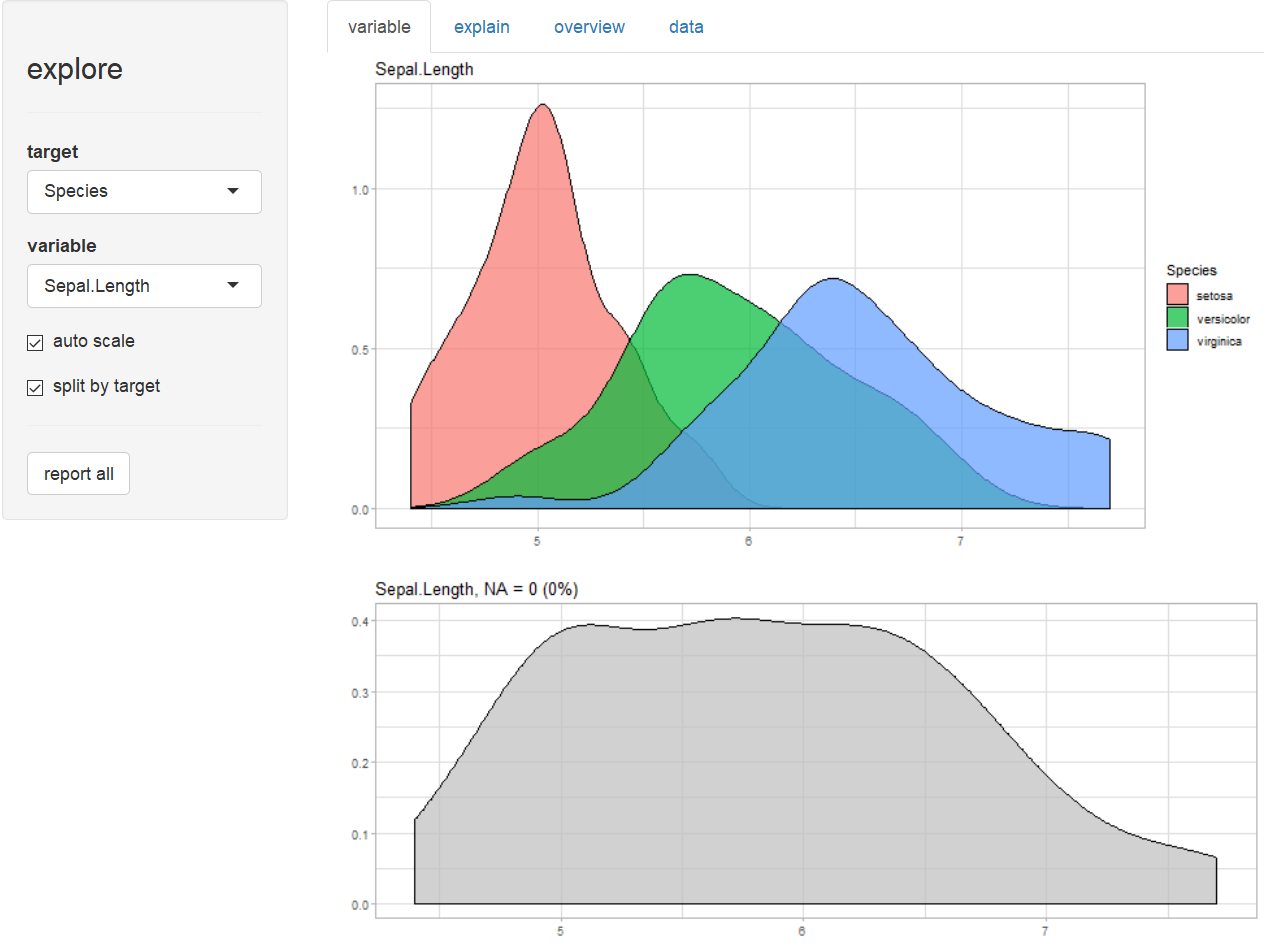You can choose each variable containng as a target, that is binary (0/1, FALSE/TRUE or “no”/“yes”), categorical or numeric.

Report variables

Create a rich HTML report of all variables with one line of code:

# report of all variables
iris %>% report(output_file = "report.html", output_dir = tempdir())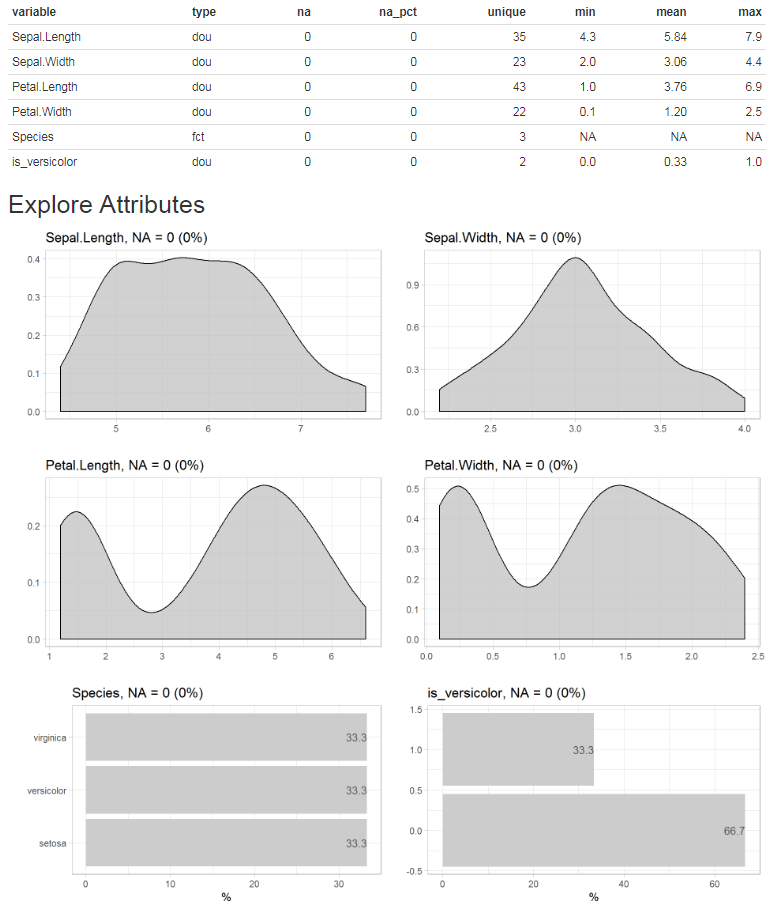Or you can simply add a target and create the report. In this case we use a binary tharget, but a categorical or numerical target would work as well.

# report of all variables and their relationship with a binary target
iris$is_versicolor <- ifelse(iris$Species == "versicolor", 1, 0)
iris %>%
report(output_file = "report.html",
output_dir = tempdir(),
target = is_versicolor)

If you use a binary tharget, the parameter split = FALSE will give you a different view on the data.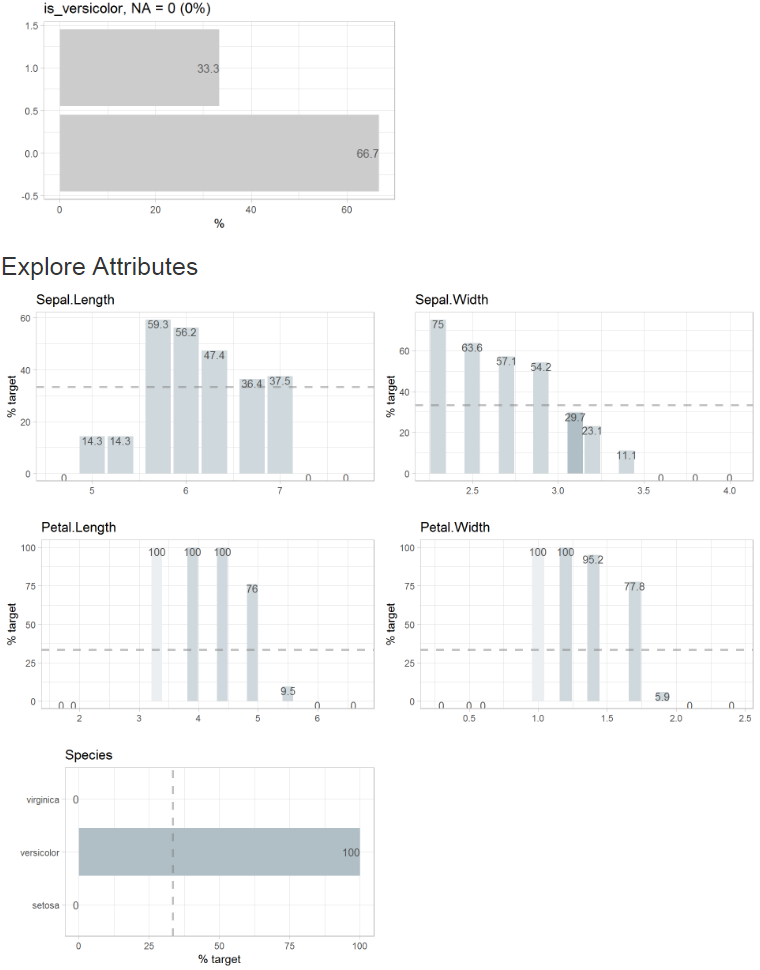Grow a decision tree

Grow a decision tree with one line of code:

iris %>% explain_tree(target = Species)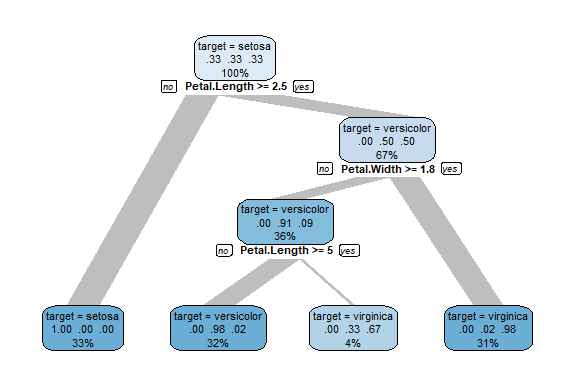You can grow a decision tree with a binary target too.

iris$is_versicolor <- ifelse(iris$Species == "versicolor", 1, 0)
iris %>% select(-Species) %>% explain_tree(target = is_versicolor)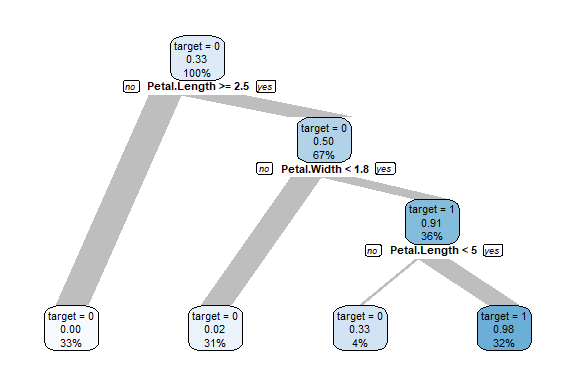Or using a numerical target. The syntax stays the same.

iris %>% explain_tree(target = Sepal.Length)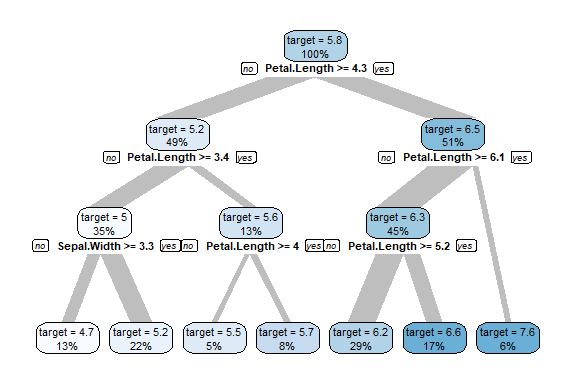You can control the growth of the tree using the parameters maxdepth, minsplit and cp.

Explore dataset

Explore your table with one line of code to see which type of variables it contains.

iris %>% explore_tbl()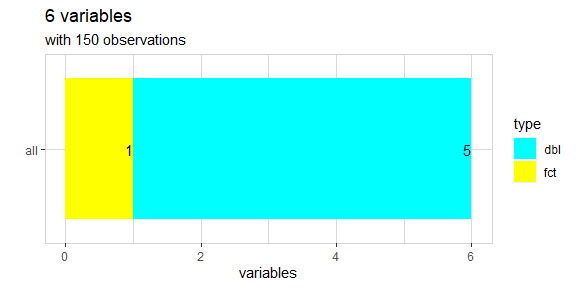You can also use describe_tbl() if you just need the main facts without visualisation.

iris %>% describe_tbl()
#> 150 observations with 6 variables
#> 0 observations containing missings (NA)
#> 0 variables containing missings (NA)
#> 0 variables with no variance

Explore variables

Explore a variable with one line of code. You don’t have to care if a variable is numerical or categorical.

iris %>% explore(Species)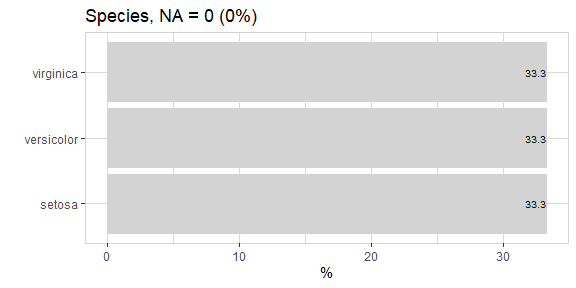iris %>% explore(Sepal.Length)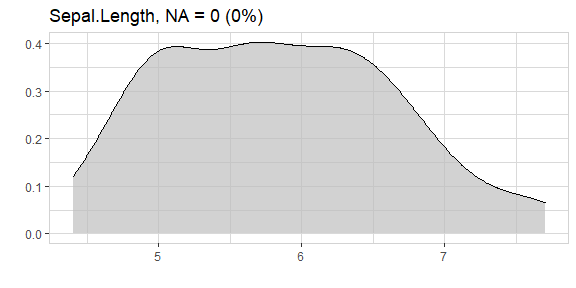Explore variables with a target

Explore a variable and its relationship with a binary target with one line of code. You don’t have to care if a variable is numerical or categorical.

iris %>% explore(Sepal.Length, target = is_versicolor)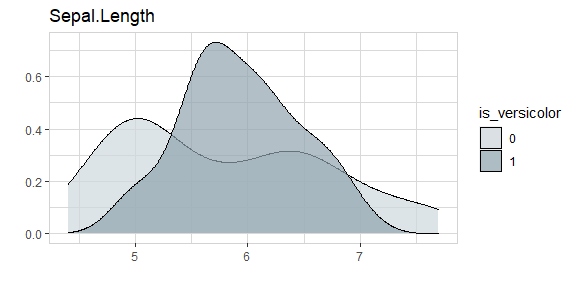Using split = FALSE will change the plot to %target:

iris %>% explore(Sepal.Length, target = is_versicolor, split = FALSE)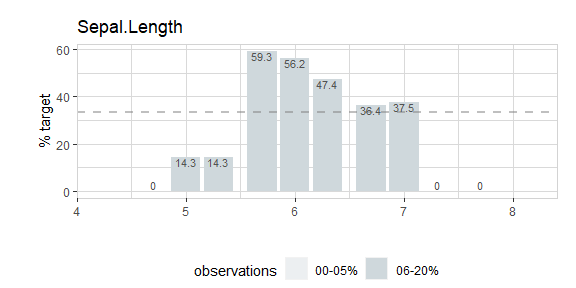The target can have more than two levels:

iris %>% explore(Sepal.Length, target = Species)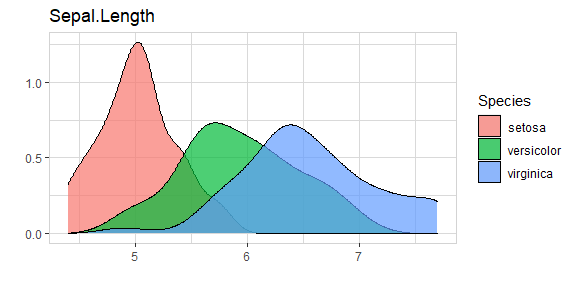Or the target can even be numeric:

iris %>% explore(Sepal.Length, target = Petal.Length)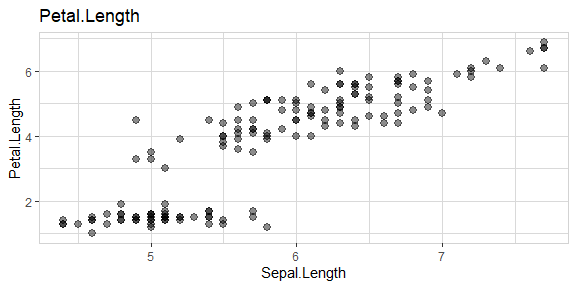Explore multiple variables

iris %>%
select(Sepal.Length, Sepal.Width) %>%
explore_all()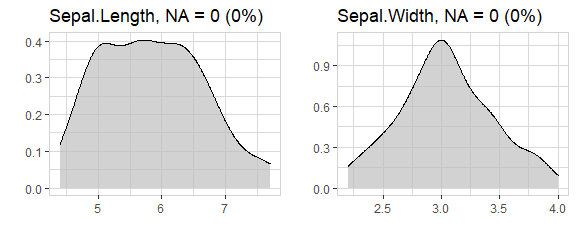iris %>%
select(Sepal.Length, Sepal.Width, is_versicolor) %>%
explore_all(target = is_versicolor)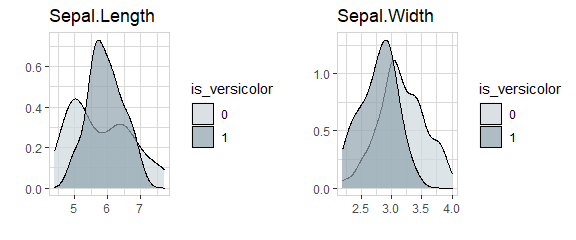iris %>%
select(Sepal.Length, Sepal.Width, is_versicolor) %>%
explore_all(target = is_versicolor, split = FALSE)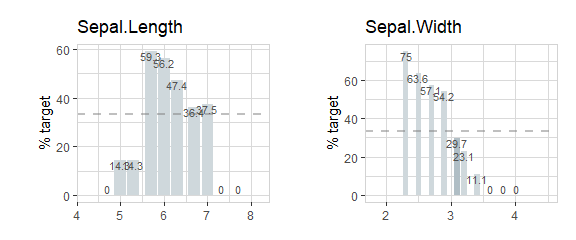iris %>%
select(Sepal.Length, Sepal.Width, Species) %>%
explore_all(target = Species)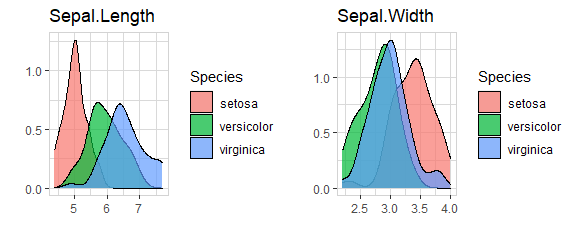iris %>%
select(Sepal.Length, Sepal.Width, Petal.Length) %>%
explore_all(target = Petal.Length)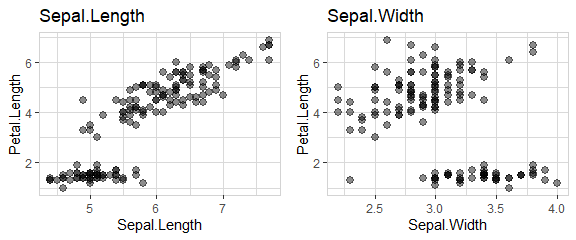data(iris)

To use a high number of variables with explore_all() in a RMarkdown-File, it is necessary to set a meaningful fig.width and fig.height in the junk. The function total_fig_height() helps to automatically set fig.height: fig.height=total_fig_height(iris)

iris %>%
explore_all()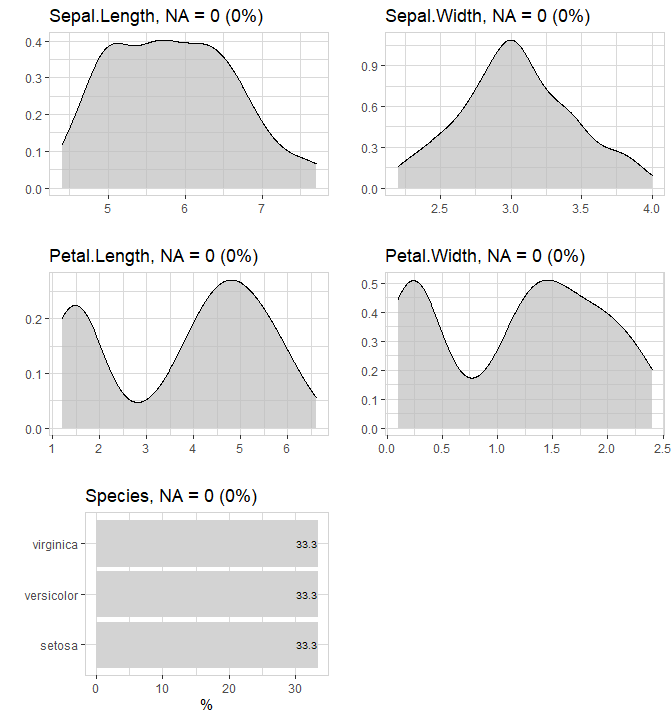If you use a target: fig.height=total_fig_height(iris, target = Species)

iris %>% explore_all(target = Species)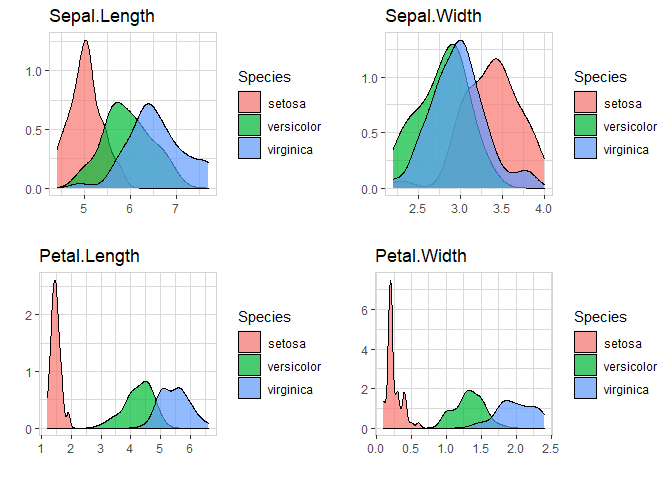You can control total_fig_height() by parameters ncols (number of columns of the plots) and size (height of 1 plot)

Explore correlation between two variables

Explore correlation between two variables with one line of code:

iris %>% explore(Sepal.Length, Petal.Length)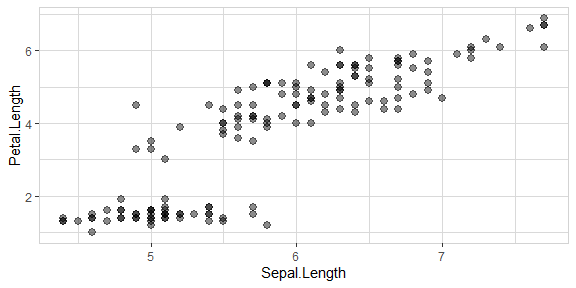You can add a target too:

iris %>% explore(Sepal.Length, Petal.Length, target = Species)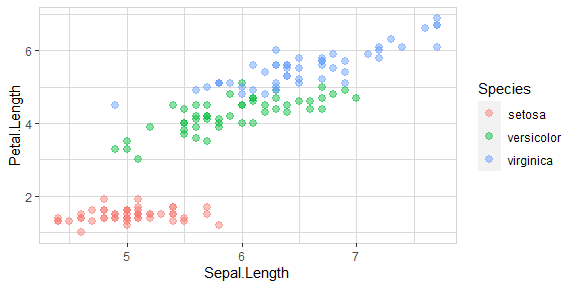Other options

If you use explore to explore a variable and want to set lower and upper limits for values, you can use the min_val and max_val parameters. All values below min_val will be set to min_val. All values above max_val will be set to max_val.

iris %>% explore(Sepal.Length, min_val = 4.5, max_val = 7)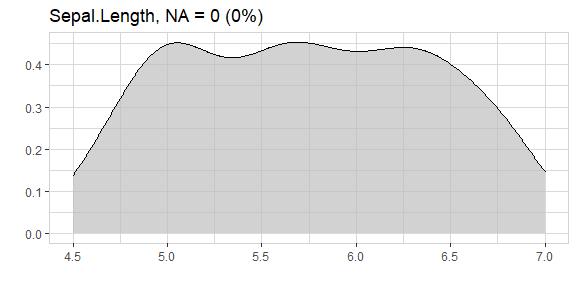explore uses auto-scale by default. To deactivate it use the parameter auto_scale = FALSE

iris %>% explore(Sepal.Length, auto_scale = FALSE)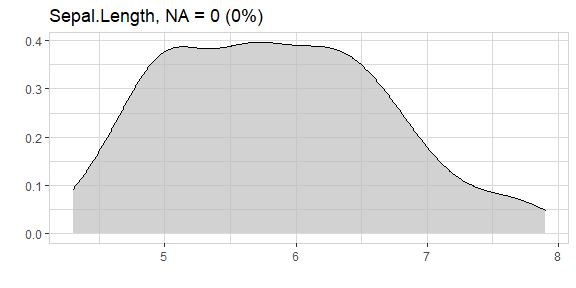Describing data

Describe your data in one line of code:

iris %>% describe()
#> # A tibble: 5 x 8
#>   variable     type     na na_pct unique   min  mean   max
#>   <chr>        <chr> <int>  <dbl>  <int> <dbl> <dbl> <dbl>
#> 1 Sepal.Length dbl       0      0     35   4.3  5.84   7.9
#> 2 Sepal.Width  dbl       0      0     23   2    3.06   4.4
#> 3 Petal.Length dbl       0      0     43   1    3.76   6.9
#> 4 Petal.Width  dbl       0      0     22   0.1  1.2    2.5
#> 5 Species      fct       0      0      3  NA   NA     NA

The result is a data-frame, where each row is a variable of your data. You can use filter from dplyr for quick checks:

# show all variables that contain less than 5 unique values
iris %>% describe() %>% filter(unique < 5)
#> # A tibble: 1 x 8
#>   variable type     na na_pct unique   min  mean   max
#>   <chr>    <chr> <int>  <dbl>  <int> <dbl> <dbl> <dbl>
#> 1 Species  fct       0      0      3    NA    NA    NA
# show all variables contain NA values
iris %>% describe() %>% filter(na > 0)
#> # A tibble: 0 x 8
#> # ... with 8 variables: variable <chr>, type <chr>, na <int>, na_pct <dbl>,
#> #   unique <int>, min <dbl>, mean <dbl>, max <dbl>

You can use describe for describing variables too. You don’t need to care if a variale is numerical or categorical. The output is a text.

# describe a numerical variable
iris %>% describe(Species)
#> variable = Species
#> type     = factor
#> na       = 0 of 150 (0%)
#> unique   = 3
#>  setosa     = 50 (33.3%)
#>  versicolor = 50 (33.3%)
#>  virginica  = 50 (33.3%)
# describe a categorical variable
iris %>% describe(Sepal.Length)
#> variable = Sepal.Length
#> type     = double
#> na       = 0 of 150 (0%)
#> unique   = 35
#> min|max  = 4.3 | 7.9
#> q05|q95  = 4.6 | 7.255
#> q25|q75  = 5.1 | 6.4
#> median   = 5.8
#> mean     = 5.843333

Data Dictionary

Create a Data Dictionary of a dataset (Markdown File data_dict.md)

iris %>% data_dict_md(output_dir = tempdir())

Add title, detailed descriptions and change default filename

description <- data.frame(
variable = c("Species"),
description = c("Species of Iris flower"))
data_dict_md(iris,
title = "iris flower data set",
description =  description,
output_file = "data_dict_iris.md",
output_dir = tempdir())

Basic data cleaning

To clean a variable you can use clean_var. With one line of code you can rename a variable, replace NA-values and set a minimum and maximum for the value.

iris %>%
clean_var(Sepal.Length,
min_val = 4.5,
max_val = 7.0,
na = 5.8,
name = "sepal_length") %>%
describe()
#> # A tibble: 5 x 8
#>   variable     type     na na_pct unique   min  mean   max
#>   <chr>        <chr> <int>  <dbl>  <int> <dbl> <dbl> <dbl>
#> 1 sepal_length dbl       0      0     26   4.5  5.81   7
#> 2 Sepal.Width  dbl       0      0     23   2    3.06   4.4
#> 3 Petal.Length dbl       0      0     43   1    3.76   6.9
#> 4 Petal.Width  dbl       0      0     22   0.1  1.2    2.5
#> 5 Species      fct       0      0      3  NA   NA     NA

Connecting to a datawarehouse

The explore package comes with a set easy to remember function to connect, read and write from/to a datawarehouse (dwh) using odbc.

# connect to a dwh(odbc DSN must be defined)
dwh <- dwh_connect("DWH_DSN")

# if you need to pass user and password
dwh <- dwh_connect("DWH_DSN",
user = "myuser",
)

# read table from a dwh